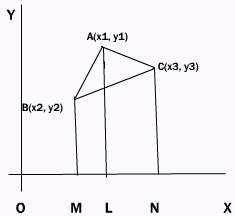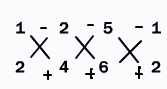Email us to get an instant 20% discount on highly effective K-12 Math & English kwizNET Programs!

#### Online Quiz (WorksheetABCD)

Questions Per Quiz = 2 4 6 8 10

### High School Mathematics - 2 Area of a triangle

 To find the area of triangle whose vertices are given.Let A(x1, y1), B(x2, y2) and C(x3, y3) be the vertices of the triangle. From A,B,C draw the perpendiculars AL, BM and CN on the x axis. Area of triangle ABC = area of trap ABML + trap ALNC - trap BMNC = 1/2[MB+LA]ML + 1/2[LA+CN]LN - 1/2[MB+NC]MN = 1/2(y2+y1) (x1-x2) + 1/2(y1+y3)(x3-x1) - 1/2(y2+y3)(x3-x2) = 1/2[x1(y2-y3)+x2(y3-y1)+x3(y1-y2)] Alternatively 1/2Example: Find the area of triangle whose vertices are (1,3), (2,4) and (5,6) Write out the x terms and y terms as shown. Multiply as shown. Divide the result by 2.1/2[1x4-2x3+2x6-5x4+5x3-1x6] = -1/2 Changing the sign, the area of the triangle in magnitude is 1/2. Directions: Solve the following.